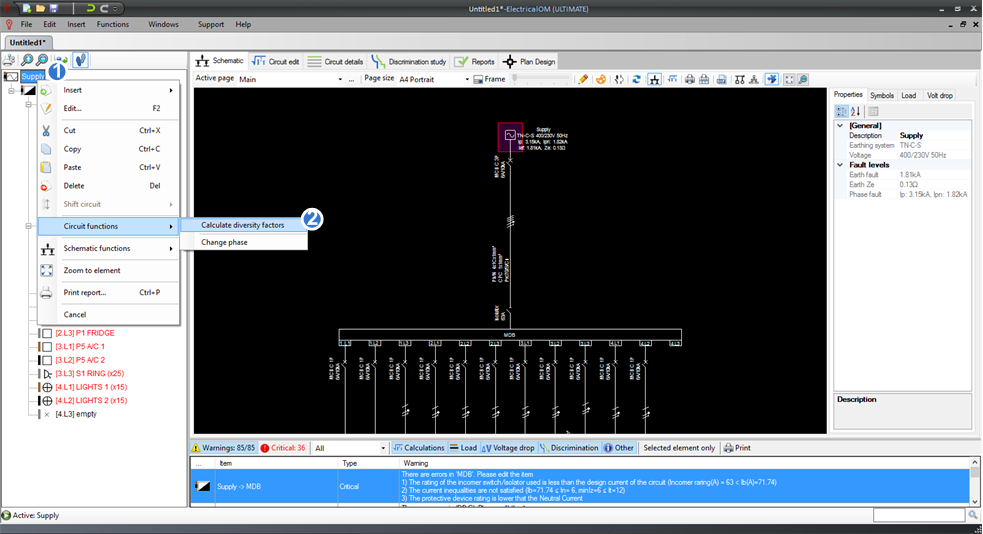At this point we have completed the first step of the work flow. The next step is to calculate the maximum demand and apply load balancing. To do this we will first apply the default diversity factors for each distribution circuit. This can be done from Circuit functions after right clicking on the Main Supply as shown below. This will calculate the diversity factors for all final circuits.We can also apply extra diversity per distribution circuit and set spare load from the circuit editor. For this tutorial we will apply an extra diversity factor of 0.9 (1) only for the Main Distribution Circuit as shown below: